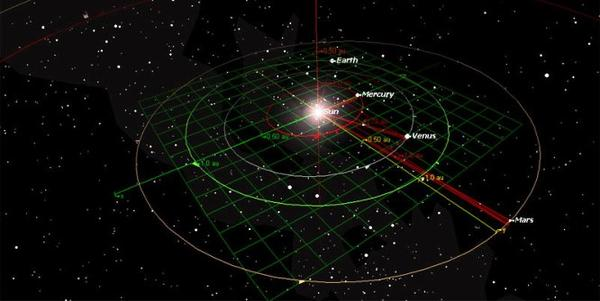# Equation of the orbital velocity of a planet

## v = 2πr/TAutomatic translation Updated March 24, 2023

The equation for calculating the speed of rotation of a planet around the sun is a mathematical formula which makes it possible to determine the speed at which a planet orbits around its star. This speed is usually expressed in terms of the orbital period, i.e. the time required for a planet to make a complete revolution around the sun.
The basic equation used to calculate the speed of rotation of a planet around the sun is: v = 2πr/T
v (in km/s) is the orbital speed, r (in km) is the average distance from the planet to the sun (average orbital radius) and T (in seconds) is the orbital period.
The constant π (pi) is a mathematical number that represents the relationship between the diameter and the circumference of a circle. It is usually rounded up to 3.14.
Using this formula, it is possible to calculate the rotational speed of all the planets of the solar system. For example, the Earth has an average distance of about 149.6 million kilometers and an orbital period of about 365.25 days (=365.25*86400 in seconds), which gives an average orbital speed of about 29.79 km/s.
Mars has an average distance of about 227.94 million kilometers and an orbital period of about 779.804 days (=779.804*86400 in seconds), giving an average orbital speed of about 21.26 km/s.

Highly elliptical orbits require more complex calculations to determine the average orbital velocity. Although it is applicable to most planets, it should be noted that it does not take into account all the factors that can influence the orbital speed of celestial bodies.

What is v = 2πr/T for?
Knowing the orbital speed of a planet is important for several reasons:
The orbital speed is directly related to the orbital period of a planet, that is to say the time it takes to make a complete orbit around its star (one year for the Earth).
It also makes it possible to calculate the distance at which it is from its host star and in particular if a planet is located in the habitable zone of its star (search for habitable planets).
A planet's orbital speed is also related to its gravity and mass. This helps to better understand the internal structure of the planet and its interaction with other planets.
It also helps to predict planetary conjunctions, eclipses, transits and other astronomical phenomena that occur in the planetary system.### Image: Inner orbits of the solar system.

1997 © Astronoo.com − Astronomy, Astrophysics, Evolution and Ecology.
"The data available on this site may be used provided that the source is duly acknowledged."